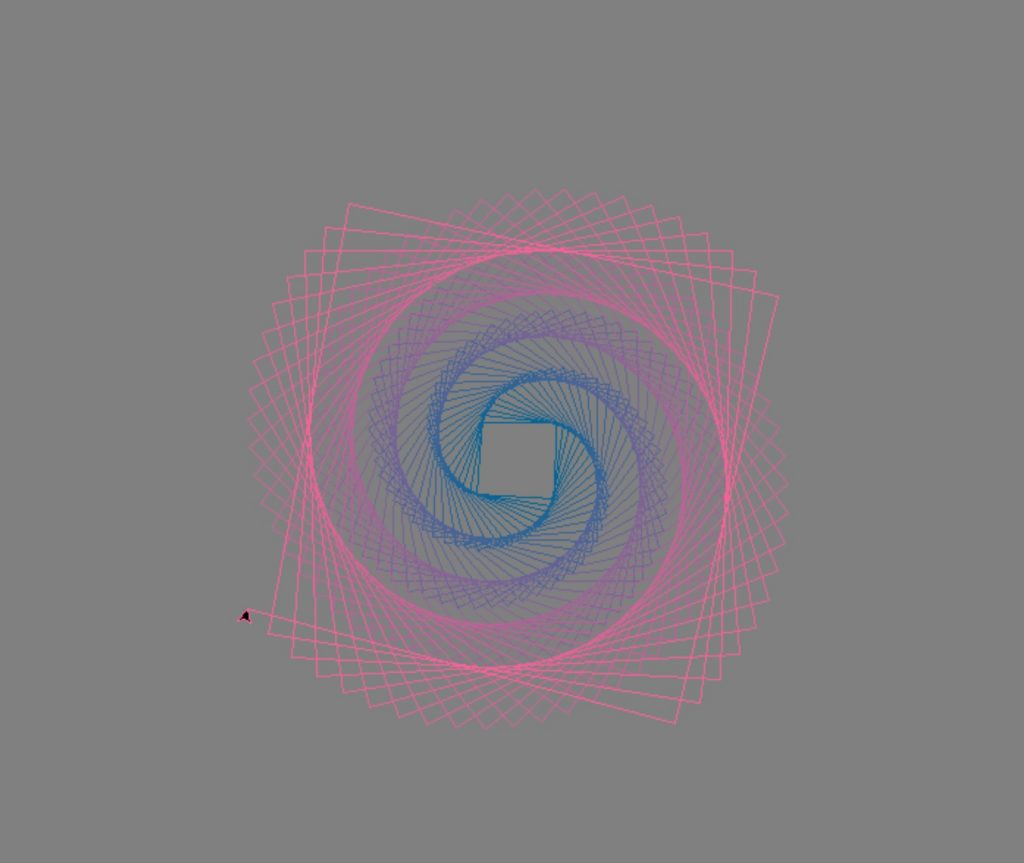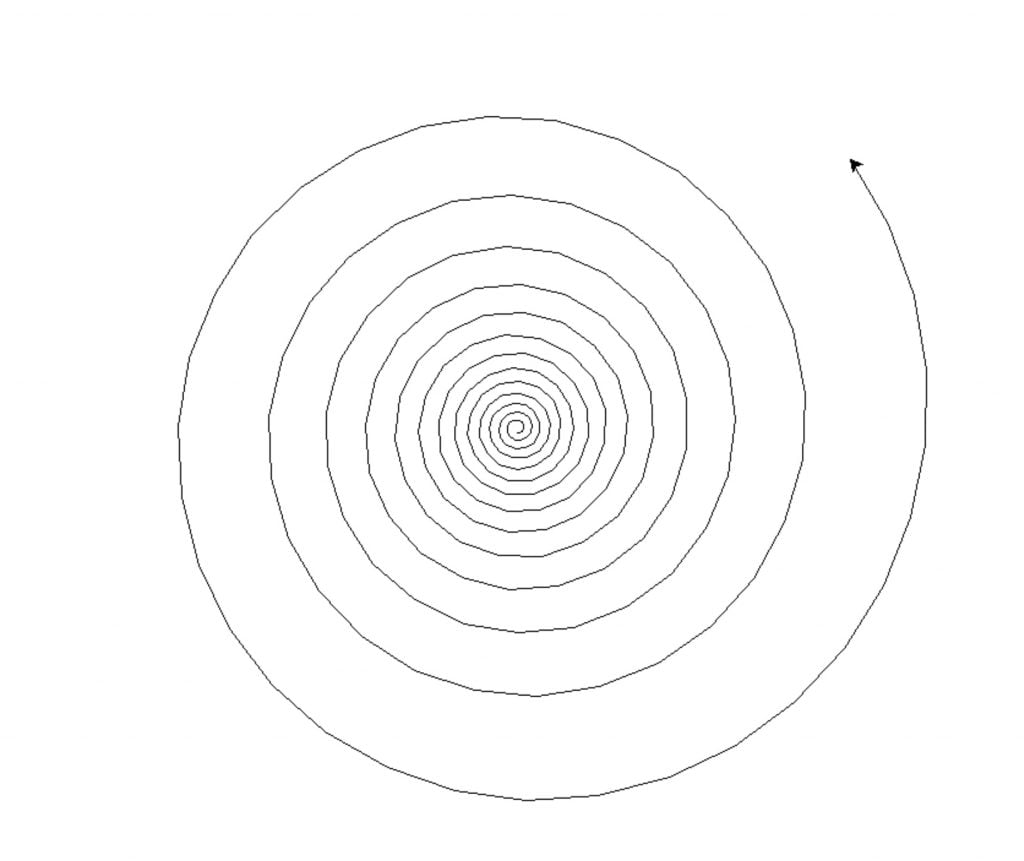## Drawing Rotating Rectangles

Here is another Python turtle example showing how to edit the background color of turtle screen and how to use RGB mode.

This example can give you a good understanding of RGB channels in images.

Here is a simply code that uses requests, json and webbrowser libraries:

``````import turtle
import random

x = 1
SN = turtle.Screen()
SN.bgcolor("gray")
a = turtle.Turtle()
a.speed(100)

i = 0
while x < 256:

r = random.randint(0,255)
g = 100
b = 150

turtle.colormode(255)
a.pencolor(i,g,b)
if x<252:
a.pensize(1)
a.forward(50 + x)
a.right(91.5)

x = x+1
i = (i+1)%255

turtle.done()   ``````Code below will print a bunch of other information regarding cocktails such as: Instructions and Ingredients and it will also open the cocktail image in a browser.

``````import turtle

a = turtle.Turtle()
for i in range(240):
a.forward(2+i/4)
a.left(30-i/12)

turtle.done()``````If you need a refresher on Python Turtle, here is a link to our Python Turtle lesson.